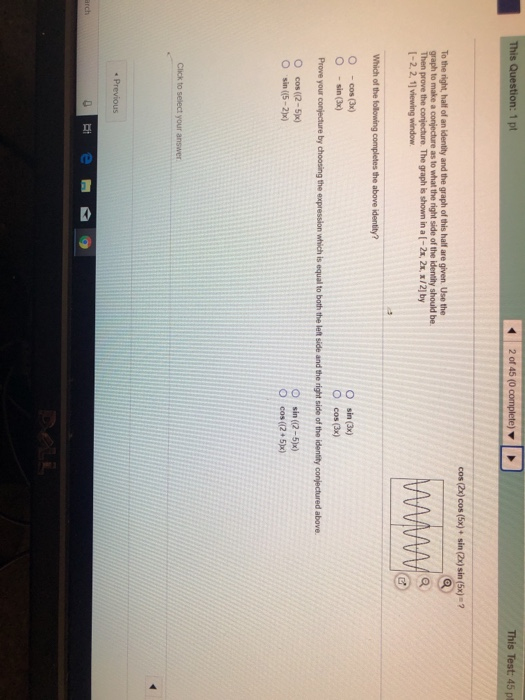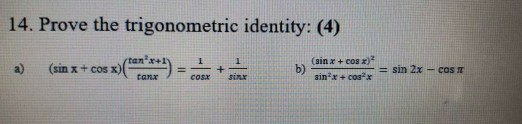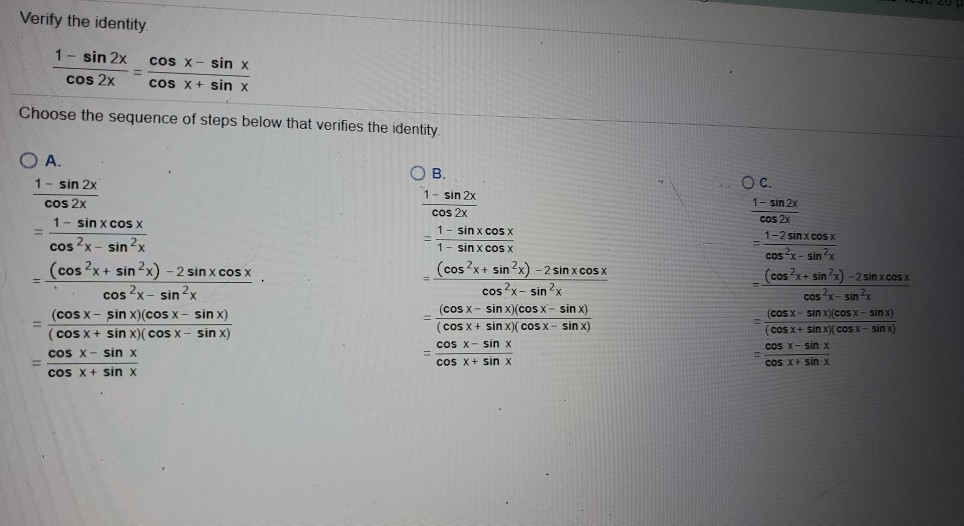# The identity of: sin^4x+cos^4x= (sin^2x+cos^2x)(sin^2x+cos^2x) sin^2x+cos^2=1 This is the answer I come up with, it is not one of the options available as an answer

The identity of:
sin^4x+cos^4x=
(sin^2x+cos^2x)(sin^2x+cos^2x)
sin^2x+cos^2=1
This is the answer I come up with, it is not one of the options available as an answer.
1. -2sin^2xcos^2x
2. 1+2sin^2x-2sin^4x
3. 1+3sin^3x-2sin^2x
4. 1-2sin^2x+2sin^4x
5. 0

check your factoring... y=sin^4x+cos^4x ... subtracting 2sin^4x ...y-2sin^4x=cos^4x-sin^4x ... factoring ...y-2sin^4x=(cos^2x+sin^2x)(cos^2x-sin^2x)=cos^2x-sin^2x ... adding 2sin^2x ...y-2sin^4x+2sin^2x=cos^2x+sin^2x=1 ... adding (2sin^4x-2sin^2x) ...y=1+2sin^4x-2sin^2x
##### Add Answer of: The identity of: sin^4x+cos^4x= (sin^2x+cos^2x)(sin^2x+cos^2x) sin^2x+cos^2=1 This is the answer I come up with, it is not one of the options available as an answer
Similar Homework Help Questions
• ### How do you do (sin^4x - sin^2x cos^2x + cos^4x) ?

In fact, the problem was: sin^6x + cos^6x = 1-3/4 sen^2 2x But... I factor the identities but I do not remember what else to do. (sin^2x + cos^2x) (sin^4x - sen^2xcos^2x + cos^4x)

• ### prove the following identity cos^4X - sin^4X = 1-2sin^2X

prove the following identitycos^4X - sin^4X = 1-2sin^2X

• ### Prove each identity: a) 1-cos^2x=tan^2xcos^2x b) cos^2x + 2sin^2x-1 = sin^2x I also tried a question on my own: tan^2x = (1 – cos^2x)/cos^2x R

Prove each identity:a) 1-cos^2x=tan^2xcos^2xb) cos^2x + 2sin^2x-1 = sin^2xI also tried a question on my own:tan^2x = (1 – cos^2x)/cos^2xR.S.= sin^2x/cos^2x I know that the Pythagorean for that is sin^2x + cos^2x That's all I could do.

• ### 1) Verify the identity cos^4x - sin^4x = cos2x I know that this is the difference of squares so (cos^2x)^2 - (sin^2x)^2 is this correct for the first step

1) Verify the identity cos^4x - sin^4x = cos2x I know that this is the difference of squares so (cos^2x)^2 - (sin^2x)^2 is this correct for the first step?

• ### This Test: 45 pt 2 of 45 (0 s Question: 1 pt cos (2x) cos (5x)+...This Test: 45 pt 2 of 45 (0 s Question: 1 pt cos (2x) cos (5x)+ sin (2x) sin (5x)-2 To the right, half of an identity and the graph of this half are given Use the graph to make a conjecture as to what the right side of the identity should be Then prove the conjecture The graphis shown in a(-2x, 2x, x/2]by I-2,2, 1] viewing window O sin (3x) 。cos(3x) O -cos (3x) O sin(3x Prove your conjecture...

• ### 1. a) Substitute u = sin(x) to evaluate sin^2(x) cos^3(x) dx. [trig identity sin2(x)+cos2(x) = 1]....

1. a) Substitute u = sin(x) to evaluate sin^2(x) cos^3(x) dx. [trig identity sin2(x)+cos2(x) = 1]. b) Find the antiderivatives: i) sin(2x) dx ii) (cos(4x)+3x^2) dx

• ### 14. Prove the trigonometric identity: (4) 2) (sin x + cos x)() come an d sin...14. Prove the trigonometric identity: (4) 2) (sin x + cos x)() come an d sin 2x – cas e

• ### sin(3x)+cos(2x)=cos^2x(1+4sinx)-sinx(1+sinx) verify the identityi want the steps along with the answer

sin(3x)+cos(2x)=cos^2x(1+4sinx)-sinx(1+sinx) verify the identityi want the steps along with the answer

• ### Verify the identity 1 - sin 2x cos 2x cOS X - sin X COS X...Verify the identity 1 - sin 2x cos 2x cOS X - sin X COS X + sin x Choose the sequence of steps below that verifies the identity OB. OC. 1-sin 2x cOS 2x 1-2 sinxcosx OA 1 - sin 2x cos 2x 1 - sin X COS X cos2x - sin 2x (cos2x+ sin 2x) - 2 sin x cos x cos2x - sin 2x (COS X - sin x)(COS X - sin x) (COS X + sin...

• ### )Find the exact solution algebriacally, if possible: (PLEASE SHOW ALL STEPS) sin 2x - sin x = 0 2

1.)Find the exact solution algebriacally, if possible:(PLEASE SHOW ALL STEPS)sin 2x - sin x = 02.) GIVEN: sin u = 3/5, 0 < u < ï/2 Find the exact values of: sin 2u, cos 2u and tan 2u using the double-angle formulas.3.)Use the half-angle formulas to determine the exact values of sine, cosine, and tangent of the angle: 15° .4.) Use the sum-to-product formulas to find the exact value of the expression:sin 195°+ sin 105°5.) Verify the identity algebraically:(cos 4x...

Free Homework App